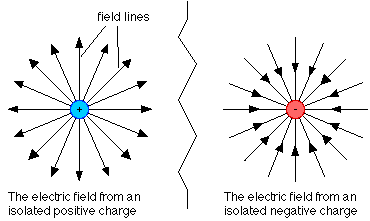# Electric Force

## Key Questions

• Electric forces are inversely proportional to the square of the distance from the source of the force.

Wow, what does that mean? In math it looks like this.

Electric Force = $\frac{{k}_{e} \cdot | {q}_{1} \cdot {q}_{2} |}{{r}^{2}}$

Where $r$ is the distance between the charges, ${q}_{1}$ and ${q}_{2}$ are the magnitudes of the two point charges, and ${k}_{e} = 8.99 \setminus \times {10}^{9} N \setminus \cdot {m}^{2} / {C}^{2}$, is a constant of proportionality called the Coulomb constant. The equation above is a simplified version of Coulomb's Law; more specifically it is the scalar form.

In simpler terms, this means the force gets four times weaker every time you double the distance. This property is known as the Inverse-Square Law. A lot of different things in physics fallow this law. For more on this check out the link below.

http://en.wikipedia.org/wiki/Inverse-square_law

• They can both be viewed as conservative vector fields.

To fully understand what that means we first have to be know what a field is. We can think of a field as a grid of arrows. Each arrow would be pointing in the direction of the force applied by the field at that spot. We can also make the arrows longer for a point with a stronger force and shorter where there is a weaker one.

Now think of a circle in the middle of our grid of arrows. Let's call this circle Earth. We know that the gravitational field always points towards the center of the Earth and that it gets weaker farther away and stronger closer. To represent this field we would need all of the arrows facing towards the origin, with longer ones close to the surface and shorter ones as we move farther away.We can now replace our Earth with an electron and the field we drew for gravity would now represent the electric field surrounding the electron. A proton would be similar, but all of the arrows would point away.Now that we know what a field is we have to define what a conservative field is. To be conservative simply means to conserve something. In the case of a field, if energy is conserved then the field is conservative. You can think of throwing a ball straight up into the air. If you throw the ball with the exact same energy it doesn't matter where you stand on the surface of our perfectly circular Earth the ball is going to go the same height. This is sometimes called path independence.

Path independence implies that it doesn't matter the path you take from point A to point B it always takes the same amount of energy. So if we pick any two points around our Earth and move from one to the other it doesn't matter what path we take. We will gain or expend the same amount of energy.In the chart above we might use a lot of energy getting the top of the hill on the blue path, but we would gain it back descending the other side. Sense both paths end at the same height then they take the same amount of energy to get there.

Friction is an example of a non-conservative force. This is because it doesn't matter what direction you travel the force is always working against you.

Below.

#### Explanation:

Electric force is something that is exerted between two particles.

$\textcolor{red}{\text{The attraction between any two bodies which either may be attractive}}$ $\textcolor{red}{\text{or repulsive is defined as an electric force}}$.

This electric force is also termed as the electrostatic force.